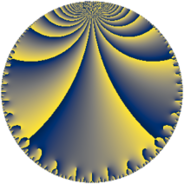# Properties

 Label 76.6.eLevel $76$ Weight $6$ Character orbit 76.e Rep. character $\chi_{76}(45,\cdot)$ Character field $\Q(\zeta_{3})$ Dimension $18$ Newform subspaces $1$ Sturm bound $60$ Trace bound $0$

# Learn more

## Defining parameters

 Level: $$N$$ $$=$$ $$76 = 2^{2} \cdot 19$$ Weight: $$k$$ $$=$$ $$6$$ Character orbit: $$[\chi]$$ $$=$$ 76.e (of order $$3$$ and degree $$2$$) Character conductor: $$\operatorname{cond}(\chi)$$ $$=$$ $$19$$ Character field: $$\Q(\zeta_{3})$$ Newform subspaces: $$1$$ Sturm bound: $$60$$ Trace bound: $$0$$

## Dimensions

The following table gives the dimensions of various subspaces of $$M_{6}(76, [\chi])$$.

Total New Old
Modular forms 106 18 88
Cusp forms 94 18 76
Eisenstein series 12 0 12

## Trace form

 $$18q - 11q^{3} + 11q^{5} + 336q^{7} - 902q^{9} + O(q^{10})$$ $$18q - 11q^{3} + 11q^{5} + 336q^{7} - 902q^{9} - 320q^{11} + 227q^{13} - 101q^{15} + 179q^{17} - 868q^{19} - 5700q^{21} - 3425q^{23} - 7054q^{25} + 14722q^{27} - 7349q^{29} - 9960q^{31} - 2998q^{33} + 15888q^{35} + 26444q^{37} - 30246q^{39} - 7311q^{41} - 8283q^{43} - 62164q^{45} + 37603q^{47} + 124738q^{49} + 47227q^{51} - 20337q^{53} + 716q^{55} - 57555q^{57} - 74455q^{59} - 7569q^{61} - 52544q^{63} + 188998q^{65} - 26177q^{67} + 116282q^{69} - 53463q^{71} - 14103q^{73} + 120912q^{75} - 31960q^{77} + 31825q^{79} - 21137q^{81} + 82600q^{83} - 50787q^{85} - 339766q^{87} - 155197q^{89} - 2800q^{91} - 46460q^{93} + 49315q^{95} + 111241q^{97} - 193544q^{99} + O(q^{100})$$

## Decomposition of $$S_{6}^{\mathrm{new}}(76, [\chi])$$ into newform subspaces

Label Dim. $$A$$ Field CM Traces $q$-expansion
$$a_2$$ $$a_3$$ $$a_5$$ $$a_7$$
76.6.e.a $$18$$ $$12.189$$ $$\mathbb{Q}[x]/(x^{18} - \cdots)$$ None $$0$$ $$-11$$ $$11$$ $$336$$ $$q+(\beta _{1}-\beta _{2}+\beta _{3})q^{3}+(\beta _{2}+\beta _{6}+\beta _{8}+\cdots)q^{5}+\cdots$$

## Decomposition of $$S_{6}^{\mathrm{old}}(76, [\chi])$$ into lower level spaces

$$S_{6}^{\mathrm{old}}(76, [\chi]) \cong$$ $$S_{6}^{\mathrm{new}}(19, [\chi])$$$$^{\oplus 3}$$$$\oplus$$$$S_{6}^{\mathrm{new}}(38, [\chi])$$$$^{\oplus 2}$$